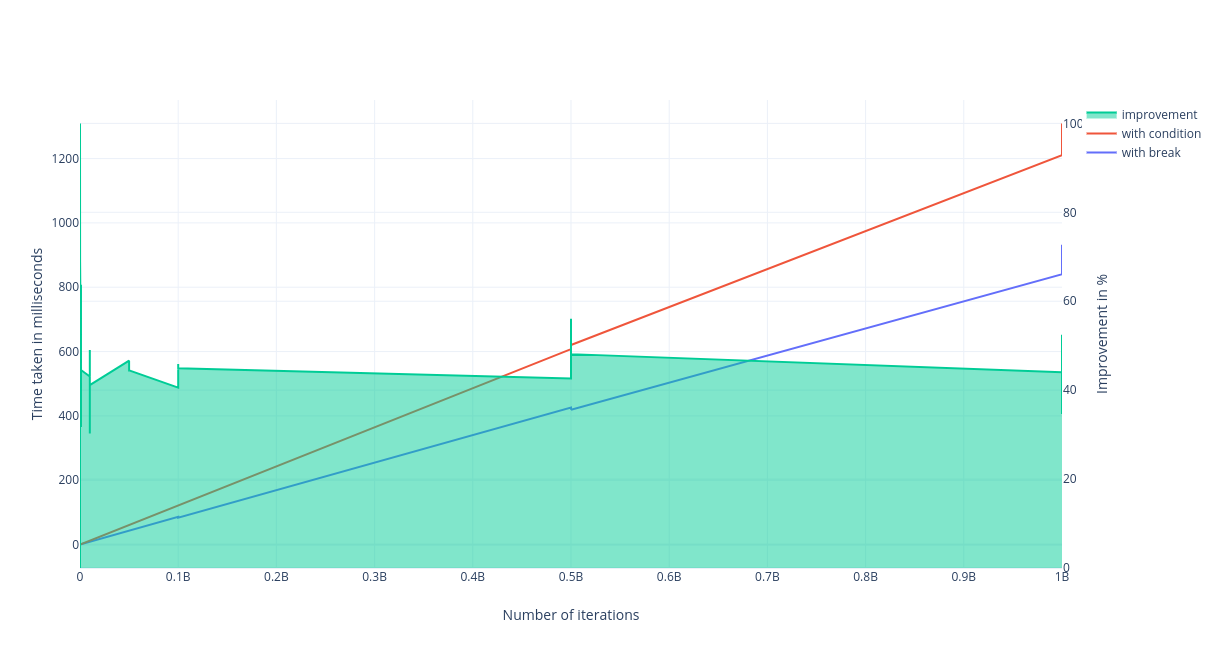# An efficient way to stop an iterating loop

Published on 6th Sep 2019

While I was writing a piece of code to solve a problem, I wrote an innocent looking for loop which I had to break when a particular condition was met. Hence wrote the most obvious looking code ever - it used `break` once condition was met and this way the flow broke out of the loop. Once my solution was accepted by an online judge I thought could I have done anything else here?

This triggered me to benchmark different ways to get out of an iterating loop. The two of the most common ways to stop iterating a loop are

• use `break` statement
• write the condition of the loop in a way that is becomes `false` when you want to stop the iteration

# The Example

Everything is better with an example. Let's say we need to find if character `a` is present in the array and we need to set the value of `bool` variable `a_found` accordingly.

### Using break statement

``````bool a_found = false;

for (int i = 0 ; i < n ; i++) {
if (str[i] == 'a') {
a_found = true;
break;
}
}
``````

### Using loop condition

``````bool a_found = false;

for (int i = 0 ; a_found == false && i < n ; i++) {
if (str[i] == 'a') {
a_found = true;
}
}
``````

Should there be considerable difference in performance? My efforts to write an article about this gives you the obvious answer. YES! there is a significant difference.

# Benchmark

The code to benchmark iterates over a string to see if `a` is present or not. The length of the string is variable and goes from 500 to 1000000000. We measure the time taken for each approach - standard benchmark practice. You can find the code here - code to benchmark stopping the loop iteration

Following graph shows the time taken by the two approaches varying with respect to the number of iterations of the loop (X Axis v/s Left Y-Axis) and the performance difference between the two approach (X Axis v/s Right Y-Axis).We see that a diverging graph that suggests one approach is significantly and always better than the other. The blue line shows the approach where we break the loop and it is always below the red one which represents the approach of stopping the iteration using condition.

We observe that using break to stop the iteration is on an average 45% better and it does not change with the number of iterations (post 1000 iterations). The raw data used for plotting this graph can be found here.

# Conclusion

Using `break` statement to break the loop is 45% better than using condition to stop the iteration and get out of the loop.## 500+ Signups

If you like what you read subscribe you can always subscribe to my newsletter and get the post delivered straight to your inbox. I write essays on various engineering topics and share it through my weekly newsletter 👇

# Other articles that you might likeFast and Efficient Pagination in MongoDB

MongoDB is a document based data store and hence pagination is one of the most common use case of it...

6th JunPseudorandom numbers using Cellular Automata - Rule 30

Generating pseudorandom numbers is an interesting problem in Computer Science. In this article, we d...

14th FebEight rituals to be a better programmer

"How to get better at programming?" is the question I had been asked quite a few times, and today I ...

28th FebSolving an age-old problem using Bayesian Average

Ranking a list of movies, products, books or even restaurants is tricky and in this article, we find...

12th Apr